## Covariance Outcome Modeling via Covariate Assisted Principal (CAP) Regression

### Xi (Rossi) LUO

The University of Texas
Health Science Center
School of Public Health
Dept of Biostatistics
and Data Science
ABCD Research GroupEcoSta 2023, Waseda University, Tokyo, Japan
August 1, 2023

Funding: NIH R01MH126970, R01EB022911; NSF/DMS 1557467

## Major CollaboratorsYi Zhao
Indiana UnivBingkai Wang
UMich BiostatChiang-shan Ray Li
Yale UnivStewart Mostofsky
Johns Hopkins MedicineBrian Caffo
Johns Hopkins Biostat

# Slides viewable on web: bit.ly/ecosta23

## Resting-state fMRI Networks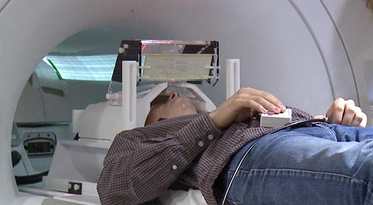• fMRI measures brain activities over time
• Resting-state: "do nothing" during scanning

• Brain networks constructed using cov/cor matrices of time series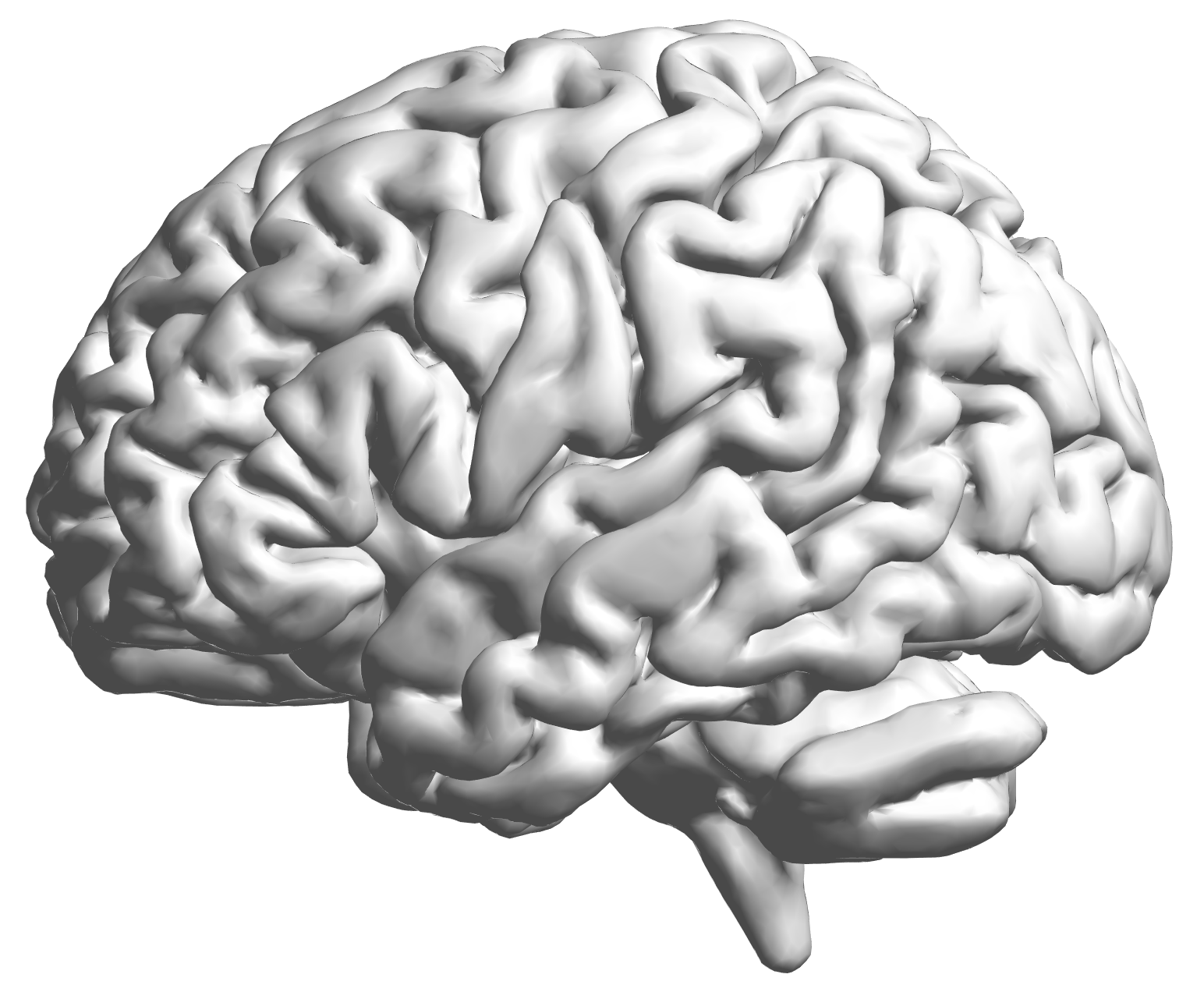fMRI data: blood-oxygen-level dependent (BOLD) signals from each cube. Each subject: observe matrix regions $\times$ time

## Brain Networks

• Brain network analysis: an emerging trend Park, Friston, Science, 2013
• Estimating brain networks from data
• Many methodological frameworks: cov, inv cov, DCM, Granger,ICA, frenquency, dynamic...
• Our group worked on graphical models Cai et al, 2001, Liu, L, 2015, DCM Cao et al, 2019
• Also on quantifying network info flow Zhao, L, 2019, Zhao L, 2021
• On utilization of networks for clustering Bunea et al, 2020
• Tree-like networks and regression Wang et al, 2022; Zhao et al, In Press
• This talk: explaining network differences

## Motivating Example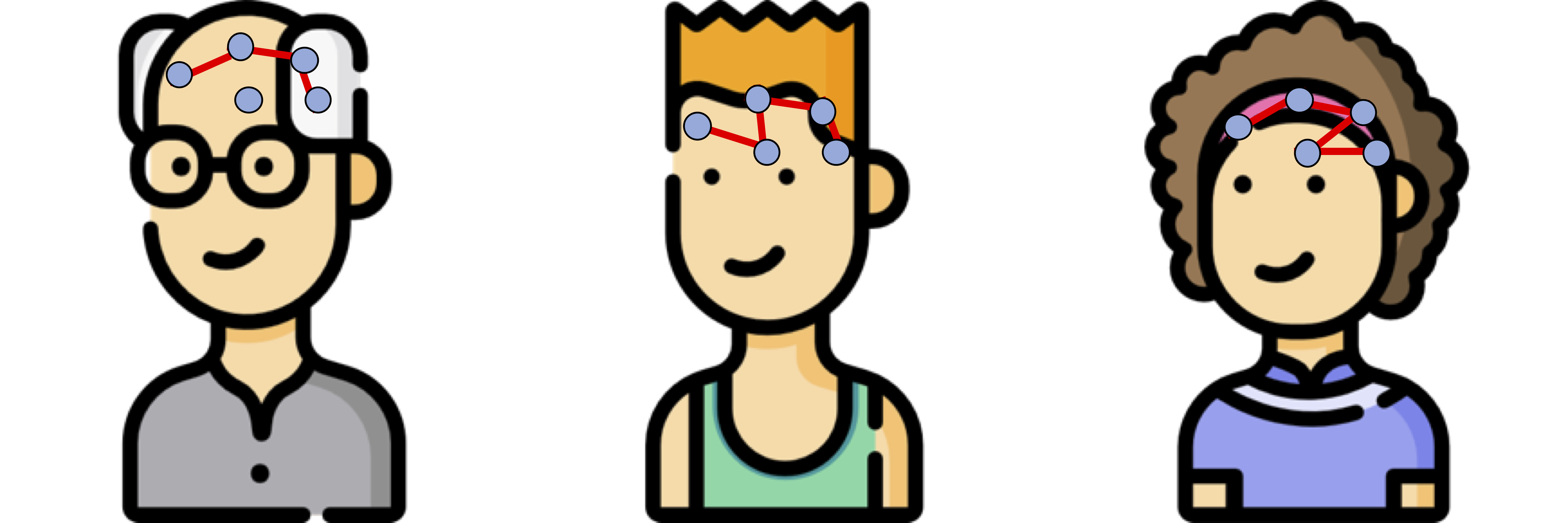Brain network connections vary by covariates (e.g. age/sex)

Goal: model how covariates modify network connections

## Our Idea

$$\textrm{function}(\textbf{graph}) = \textbf{age}\times \beta_1 + \textbf{sex}\times \beta_2 + \cdots$$

## Other Related Applications

• Differences in genetic networks
• Networks by disease/age/sex groups
• Single cell sequencing
• Covariance modeling
• Temporal changes in covariance matrices
• Spatial-temporal
• Theme: signals in the second moment, low/no signals in the first moment

## Mathematical Problem

• Given $n$ (semi-)positive matrices, $\Sigma_i\in \real^{p\times p}$
• Given $n$ corresponding vector covariates, $x_i \in \real^{q}$
• Find function $g(\Sigma_i) = x_i \beta$, $i=1,\dotsc, n$
• In essense, regress positive matrices on vectors

## Other Extensions

• Linear model formulation, and thus very flexible
• Easily extended to big data with 1 million brain regions, Brain and Behavior
• High dim cov (later), Electronic J of Stat
• Many other extensions under review or preparation: longitudinal, mixed effects, ...

## Some Related Problems

• Heterogeneous regression or weighted LS:
• Usually for scalar variance $\sigma_i$, find $g(\sigma_i) = f(x_i)$
• Goal: to improve efficiency, not to interpret $x_i \beta$
• Covariance models Anderson, 73; Pourahmadi, 99; Hoff, Niu, 12; Fox, Dunson, 15; Zou, 17
• Model $\Sigma_i = g(x_i)$, sometimes $n=i=1$
• Goal: better models for $\Sigma_i$
• Multi-group PCA, (inverse) covariance estimation/testing Flury, 84, 88; Boik 02; Hoff 09; Franks, Hoff, 16, Guo et al, 11; Tsai et al, 22; Cai et al, 13
• No regression model, cannot handle vector $x_i$
• Goal: find common/uncommon parts of multiple (inv) $\Sigma_i$
• Tensor-on-scalar regression Li, Zhang, 17; Sun, Li, 17
• No guarantees for positive matrix outcomes

# CAP for Low-dimensional Cov Outcomes

## Naive: Massive Edgewise Regressions

• Intuitive method by mostly neuroscientists
• Try $g_{j,k}(\Sigma_i) = \Sigma_{i}[j,k] = x_i \beta$
• Repeat for all $(j,k) \in \{1,\dotsc, p\}^2$ pairs
• Essentially $O(p^2)$ regressions for each connection
• Limitations:
• multiple testing $O(p^2)$
• failure to accout for dependencies between regressions
• low signal to noise ratio elementwise, spectral modeling may be more robust

## $\mbox{Modified PCA}(\Sigma_i) = x_i \beta$

• Essentially, we aim to turn unsupervised PCA to a supervised PCA
• Ours differs from existing PCA methods:
• Supervised PCA Bair et al, 06 models scalar-on-vector
• However, our CAP decomposition is almost always different from PCA

# Model and Method

## Model

• Find principal direction (PD) $\gamma \in \real^p$, such that: $$\log({\gamma}^\top\Sigma_{i}{\gamma})=\beta_{0}+x_{i}^\top{\beta}_{1}, \quad i =1,\dotsc, n$$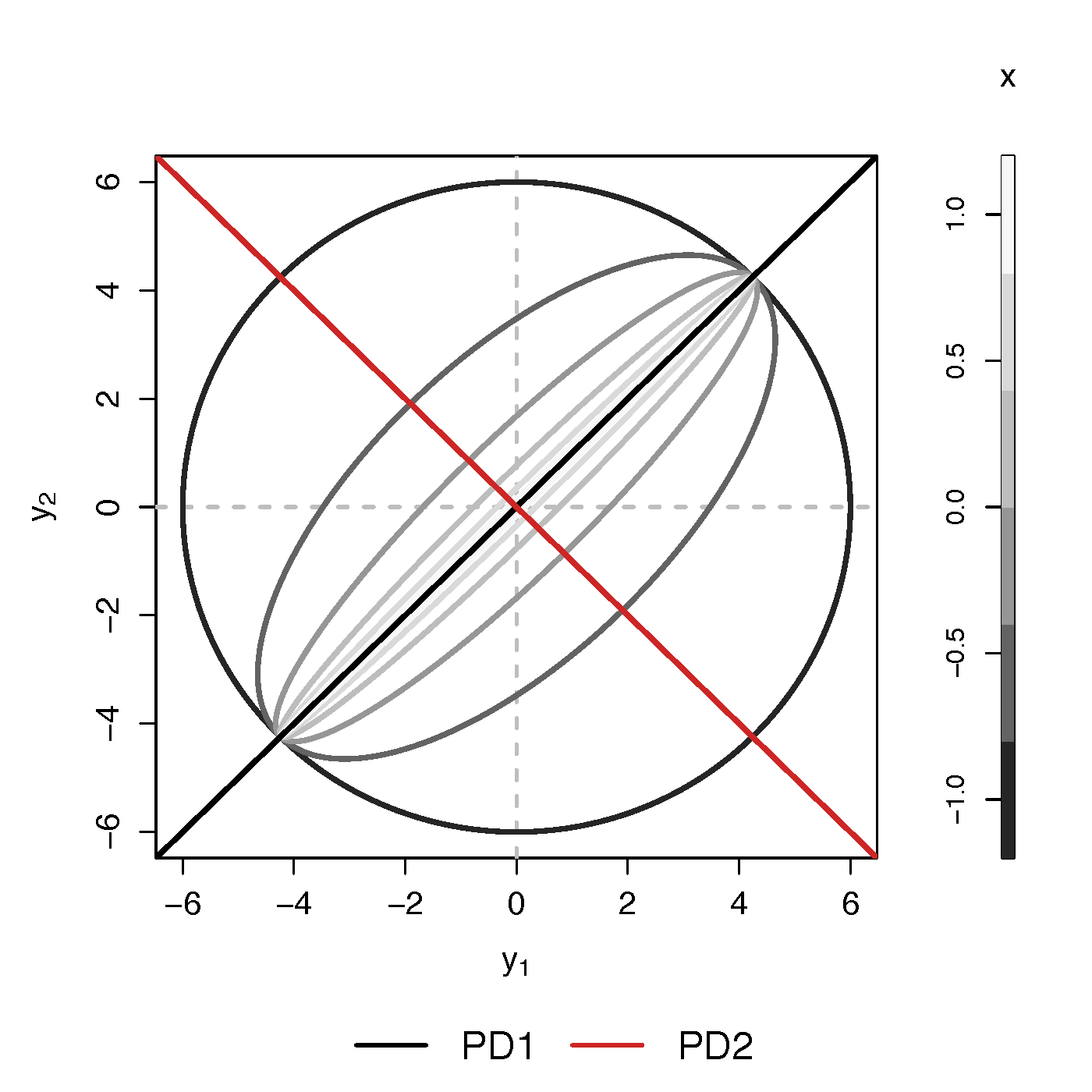Example (p=2): PD1 largest variation in $\Sigma_i$ but not related to $x$

PCA selects PD1, Ours selects PD2

• Scalability: potentially for $p \sim 10^6$ or larger
• Interpretation: covariate assisted PCA
• Turn unsupervised PCA into supervised
• Sensitivity: target those covariate-related variations
• Covariate assisted SVD?
• Potential applications in other big data problems besides fMRI

## Method

• MLE with constraints: $$\scriptsize \begin{eqnarray}\label{eq:obj_func} \underset{\boldsymbol{\beta},\boldsymbol{\gamma}}{\text{minimize}} && \ell(\boldsymbol{\beta},\boldsymbol{\gamma}) := \frac{1}{2}\sum_{i=1}^{n}(x_{i}^\top\boldsymbol{\beta}) \cdot T_{i} +\frac{1}{2}\sum_{i=1}^{n}\boldsymbol{\gamma}^\top \Sigma_{i}\boldsymbol{\gamma} \cdot \exp(-x_{i}^\top\boldsymbol{\beta}) , \nonumber \\ \text{such that} && \boldsymbol{\gamma}^\top H \boldsymbol{\gamma}=1 \end{eqnarray}$$
• No meaningful solutions without constraints
• Two obvious constriants:
• C1: $H = I$
• C2: $H = n^{-1} (\Sigma_1 + \cdots + \Sigma_n)$
• The most natural C1 usually does not work!

## Choice of $H$

Proposition: When (C1) $H=\boldsymbol{\mathrm{I}}$ in the optimization problem, for any fixed $\boldsymbol{\beta}$, the solution of $\boldsymbol{\gamma}$ is the eigenvector corresponding to the minimum eigenvalue of matrix $$\sum_{i=1}^{n}\frac{\Sigma_{i}}{\exp(x_{i}^\top\boldsymbol{\beta})}$$

• C1 leads to small eigen values (potential noises)
• Will focus on the constraint (C2)

## Algoirthm

• Iteratively update $\beta$ and then $\gamma$
• Prove explicit updates (see our papers)
• Extension to multiple $\gamma$:
• After finding $\gamma^{(1)}$, we will update $\Sigma_i$ by removing its effect
• Search for the next PD $\gamma^{(k)}$, $k=2, \dotsc$
• Impose the orthogonal constraints such that $\gamma^{k}$ is orthogonal to all $\gamma^{(t)}$ for $t\lt k$

## Theory for $\beta$

Theorem: Assume $\sum_{i=1}^{n}x_{i}x_{i}^\top/n\rightarrow Q$ as $n\rightarrow\infty$. Let $T=\min_{i}T_{i}$, $M_{n}=\sum_{i=1}^{n}T_{i}$, under the true $\boldsymbol{\gamma}$, we have \begin{equation} \sqrt{M_{n}}\left(\hat{\boldsymbol{\beta}}-\boldsymbol{\beta}\right)\overset{\mathcal{D}}{\longrightarrow}\mathcal{N}\left(\boldsymbol{\mathrm{0}},2 Q^{-1}\right),\quad \text{as } n,T\rightarrow\infty, \end{equation} where $\hat{\boldsymbol{\beta}}$ is the maximum likelihood estimator when the true $\boldsymbol{\gamma}$ is known.

## Theory for $\gamma$

Theorem: Assume $\Sigma_{i}=\Gamma\Lambda_{i}\Gamma^\top$, where $\Gamma=(\boldsymbol{\gamma}_{1},\dots,\boldsymbol{\gamma}_{p})$ is an orthogonal matrix and $\Lambda_{i}=\mathrm{diag}\{\lambda_{i1},\dots,\lambda_{ip}\}$ with $\lambda_{ik}\neq\lambda_{il}$ ($k\neq l$), for at least one $i\in\{1,\dots,n\}$. There exists $k\in\{1,\dots,p\}$ such that for $\forall~i\in\{1,\dots,n\}$, $\boldsymbol{\gamma}_{k}^\top\Sigma_{i}\boldsymbol{\gamma}_{k}=\exp(x_{i}^\top\boldsymbol{\beta})$. Let $\hat{\boldsymbol{\gamma}}$ be the maximum likelihood estimator of $\boldsymbol{\gamma}_{k}$ in Flury, 84. Then assuming that the assumptions are satisfied,

$\hat{ \boldsymbol{\beta}}$ from our algorithm is $\sqrt{M_{n}}$-consistent estimator of $\boldsymbol{\beta}$.

# Simulations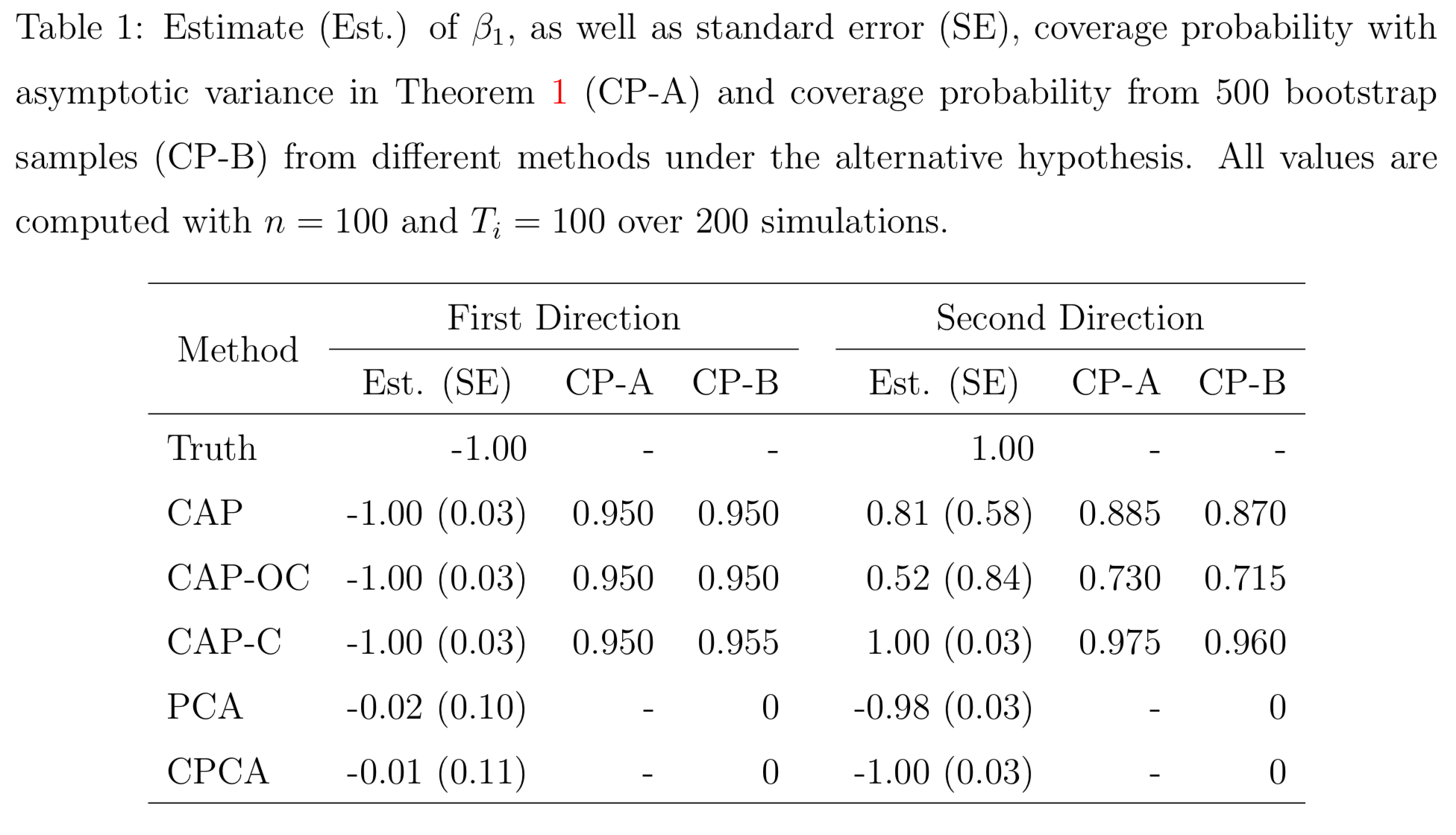PCA and common PCA do not find the first principal direction, because they don't consider covariates

# Resting-state fMRI

## Regression CoefficientsAge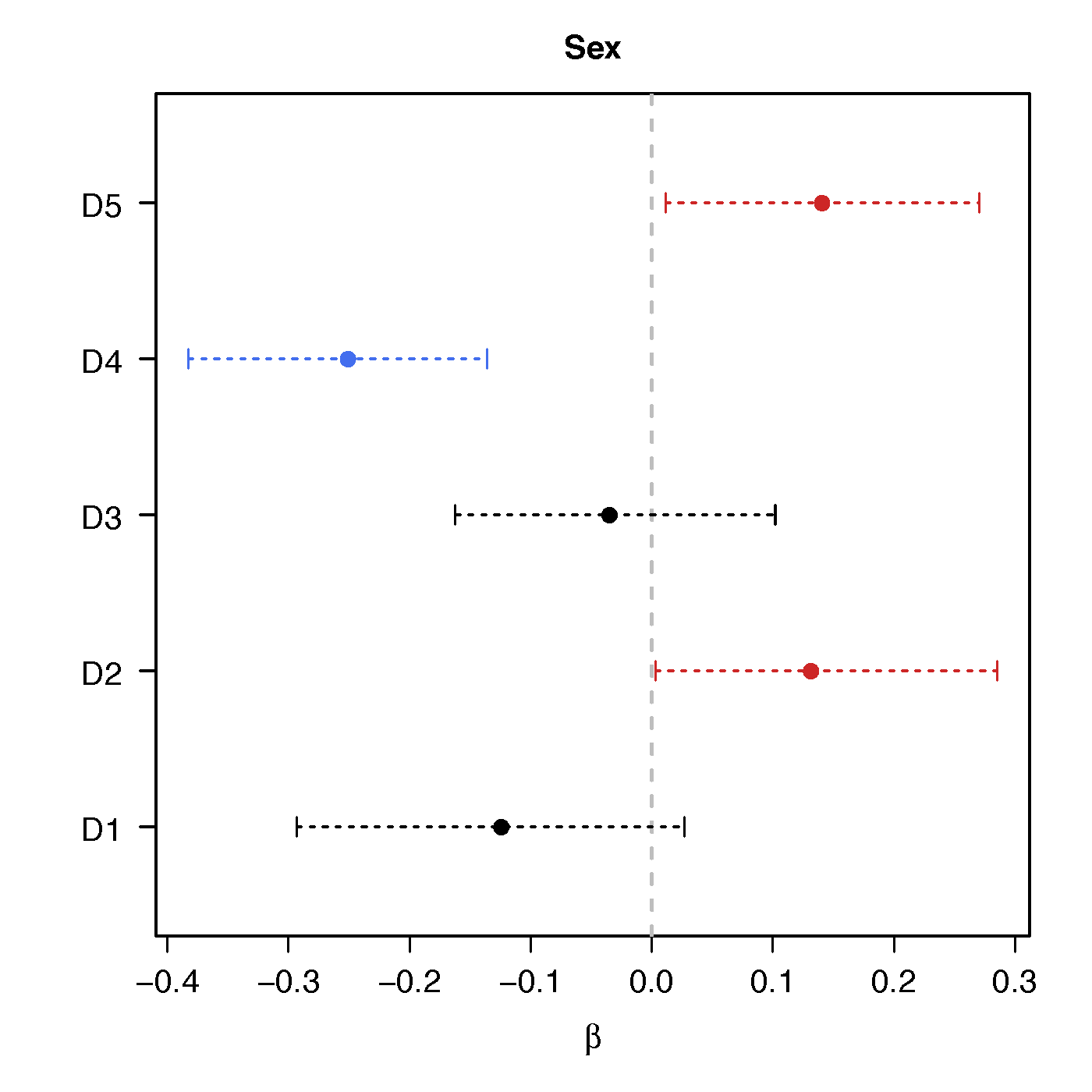Sex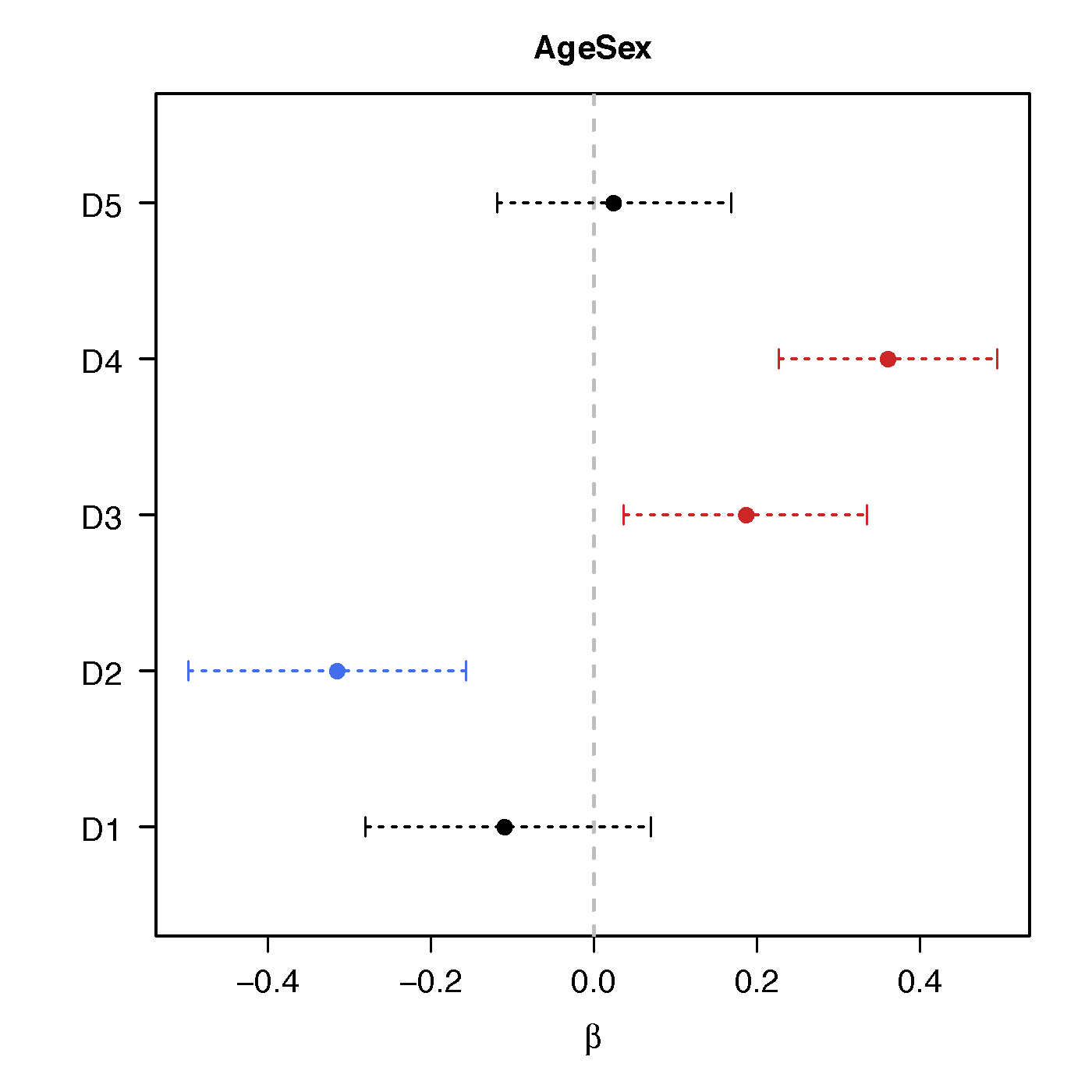Age*Sex

• Ours above: significant network differences due to age, sex and their interactions
• Naive massive edgewise regression: no statistical significant changes in cov entries

## Brain Map of $\gamma$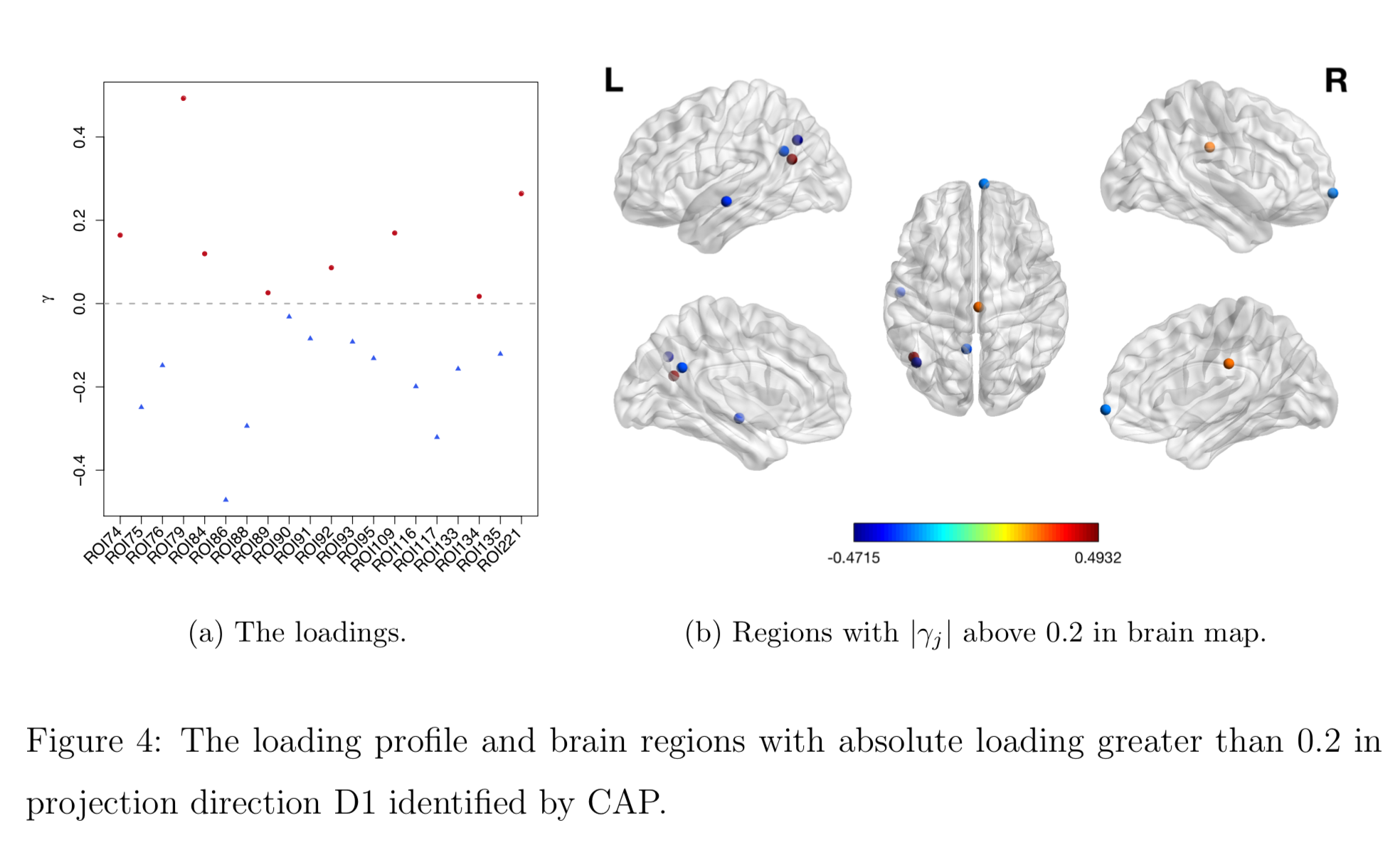## High-Dim Cov Extensions

• Voxel level (super-high dim) cov matrices Zhao et al, 2020
• Raw cov: $10^6 \times 10^6$=Trillions of cov elements
• Decompose data/networks via ICA/PCA
• Explain netowrk diff on reduced dim
• Reconstruct brain network maps at the voxel level
• High dimensional cov Zhao et al, 2021
• Joint shrinkage Ledoit, Wolf, 2004 of multiple cov
• Optimum in theory by our joint shrinkage
• Method/theory also work with joint shrinkage

# CAP for High-dimensional Cov Outcomes

## Challenges in High-dim

• Sample covariance not full rank in high-dim: when sample size $T_i$ ≪ variable size $p$
• $p$ fixed in the previous theory
• Sample cov is a poor estimator in high dim
• Eigenvalues even more dispersed
• Regularization Ledoit, Wolf 04 less optimal

## L-W Cov Shrinkage

• By Ledoit and Wolf (2004), over 2800 citations so far
• Given sample cov $S$, find estimator $\Sigma^*$ by $$\begin{eqnarray} \underset{\mu,\rho}{\text{minimize}} && \mathbb{E}\left\| \Sigma^{*}- S \right\|^{2} \nonumber \\ \text{such that} && \Sigma^{*}=\rho\mu\boldsymbol{\mathrm{I}}+(1-\rho)S \end{eqnarray}$$
• $\rho$ and $\mu$ can be estimated consistently, and thus $\Sigma^*$ consistently
• Limitations: not handling multiple covariance matrices jointly, not using the covariate info

## Our Contributions

• New joint shrinkage estimator for multiple covariances
• Incorporate covariate info in regression
• Minimum quadratic risk asymptotically among all linear combinations, while L-W is suboptimal
• Also provide consistent estimator for CAP

## Covariate Dependent CAP Shrinkage

• Propose CAP shrinkage by $$\begin{eqnarray} \underset{\mu,\rho}{\text{minimize}} && \frac{1}{n}\sum_{i=1}^{n}\mathbb{E}\left\{\gamma^\top\Sigma_{i}^{*}\gamma-\exp( x_{i}^\top\beta)\right\}^{2} \nonumber \\ \text{such that} && \Sigma_{i}^{*}=\rho\mu\boldsymbol{\mathrm{I}}+(1-\rho)S_{i}, \quad \text{for } i=1,\dots,n. \end{eqnarray}$$
• Prove a theorem for solving the optimization and an estimator for $\Sigma_i^*$ $$S_i^* = \hat{f}(\gamma, x_i, \beta) \boldsymbol{\mathrm{I}} + \hat{g}(\gamma, x_i, \beta) S_i$$ where $S_i^*$ is a consistent estimator, $\hat{f}$ and $\hat{g}$ are computed by explicit formulas from data

## CS-CAP Algorithm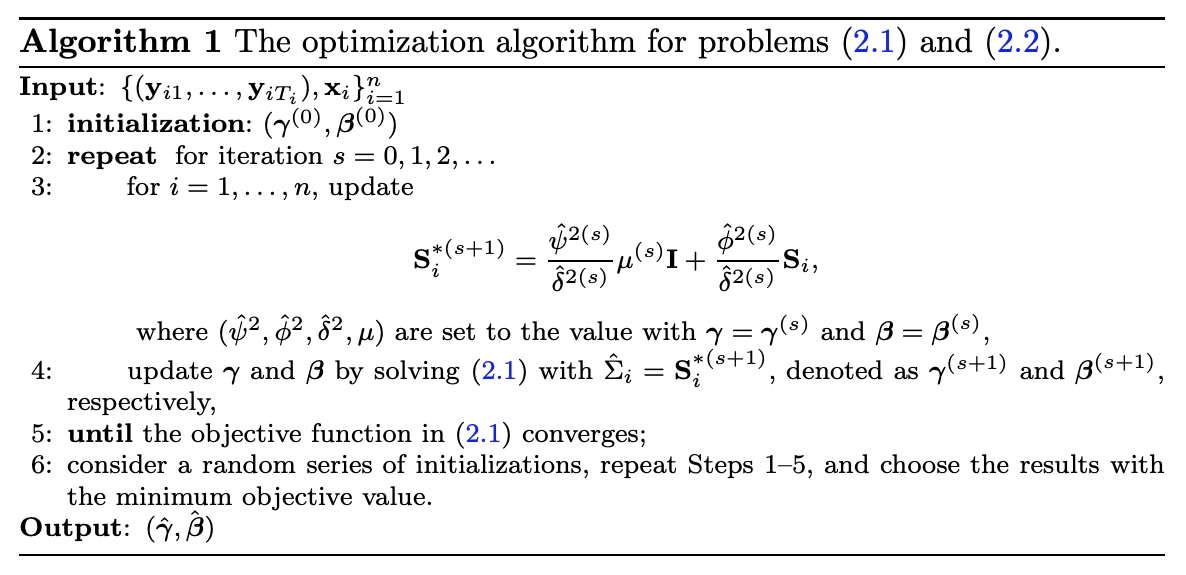Iteratively update $S^*$, $\gamma$, $\beta$ until cconvergence

Theorem: Assume $(\gamma, \beta)$ is given. With a fixed $n\in\mathbb{N}^{+}$, for any sequence of linear combinations $\{\hat{\Sigma}_{i}\}_{i=1}^{n}$ of the identity matrix and the sample covariance matrix, where the combination coefficients are constant over $i\in\{1,\dots,n\}$, the estimator $S_{i}^{*}$ verifies: $$\tiny \begin{equation} \lim_{T\rightarrow\infty}\inf_{T_{i}\geq T}\left[\frac{1}{n}\sum_{i=1}^{n}\mathbb{E}\left\{\gamma^\top\hat{\Sigma}_{i}\gamma-\exp(\bx_{i}^\top \beta)\right\}^{2}-\frac{1}{n}\sum_{i=1}^{n}\mathbb{E}\left\{\gamma^\top S_{i}^{*}\gamma-\exp(\bx_{i}^\top \beta)\right\}^{2}\right]\geq 0. \end{equation}$$ In addition, every sequence of $\{\hat{\Sigma}_{i}\}_{i=1}^{n}$ that performs as well as $\{ S_{i}^{*}\}_{i=1}^{n}$ is identical to $\{ S_{i}^{*}\}_{i=1}^{n}$ in the limit: $$\tiny \begin{equation} \lim_{T\rightarrow\infty}\left[\frac{1}{n}\sum_{i=1}^{n}\mathbb{E}\left\{\gamma^\top\hat{\Sigma}_{i}\gamma-\exp(\bx_{i}^\top \beta)\right\}^{2}-\frac{1}{n}\sum_{i=1}^{n}\mathbb{E}\left\{\gamma^\top S_{i}^{*}\gamma-\exp(\bx_{i}^\top \beta)\right\}^{2}\right]=0 \end{equation}$$ $$\scriptsize \begin{equation} \Leftrightarrow \quad \mathbb{E}\|\hat{\Sigma}_{i}- S_{i}^{*}\|^{2} \rightarrow 0, \quad \text{for } i=1,\dots,n. \end{equation}$$

Our Covariate-dependent Shrinkage CAP (CS-CAP) is optimal

## Simu: new CS-CAP vs L-W and CAP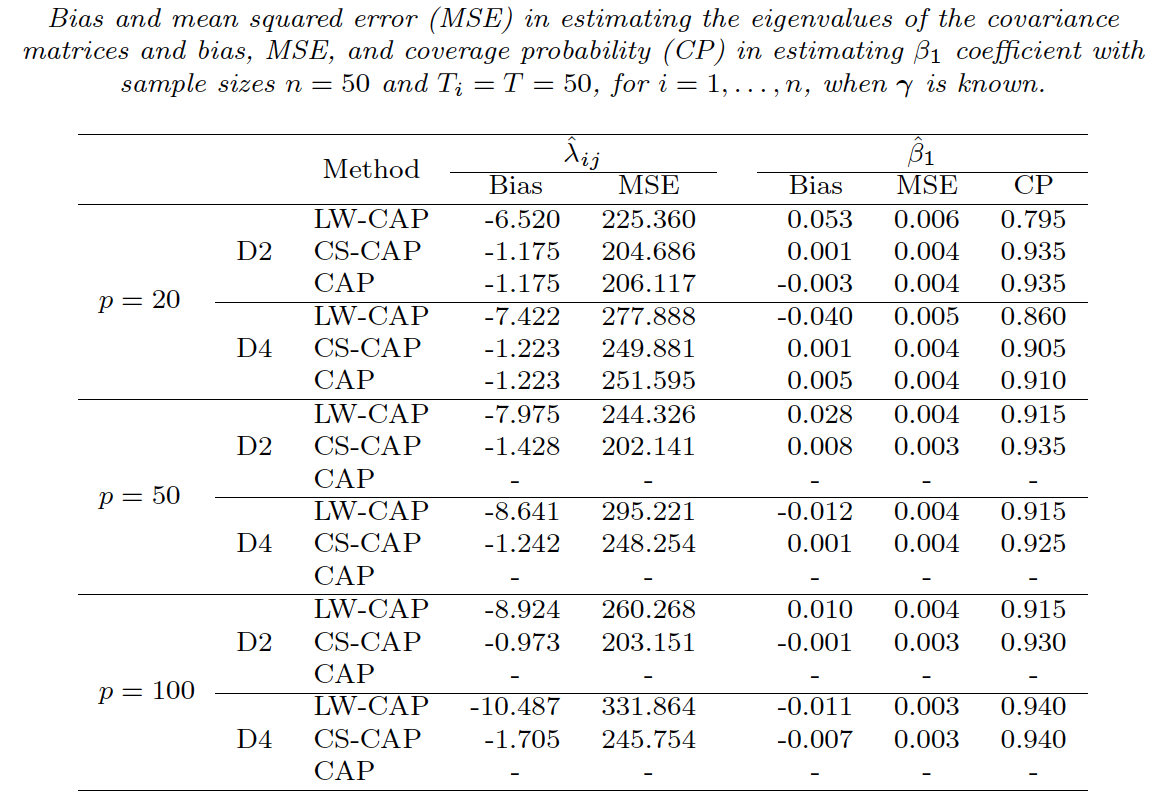• The Alzheimer’s Disease Neuroimaging Initiative (ADNI): launched in 2003, for studying ADRD
• Alzheimer's Disease Related Dementias (ADRD) affects more than 6 million US people, and 55 million worldwide
• No known* treatment to stop or prevent ADRD
• fMRI and brain connectivity likely to be interrupted prior to dementia
• APOE-$\varepsilon$4 gene, strong risk factor and potential treatment target

## Covariate Implicated Components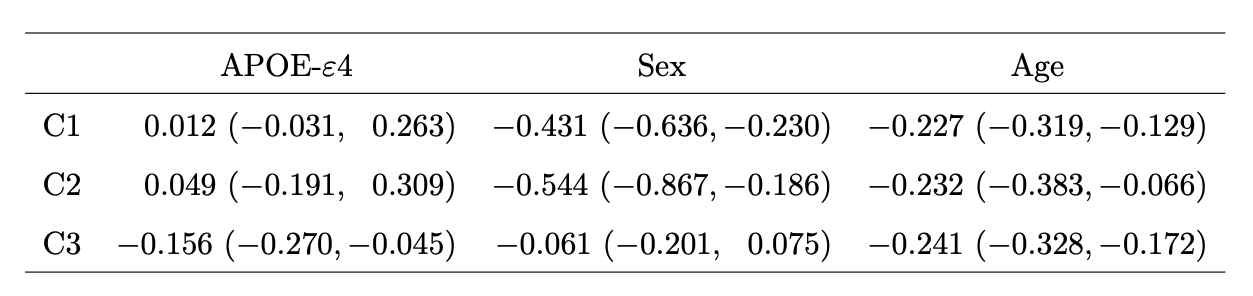• First two components related to age and sex
• Last C3 predicted by age and APOE-$\varepsilon$4 gene

## C3: APOE Areas Found by CS-CAP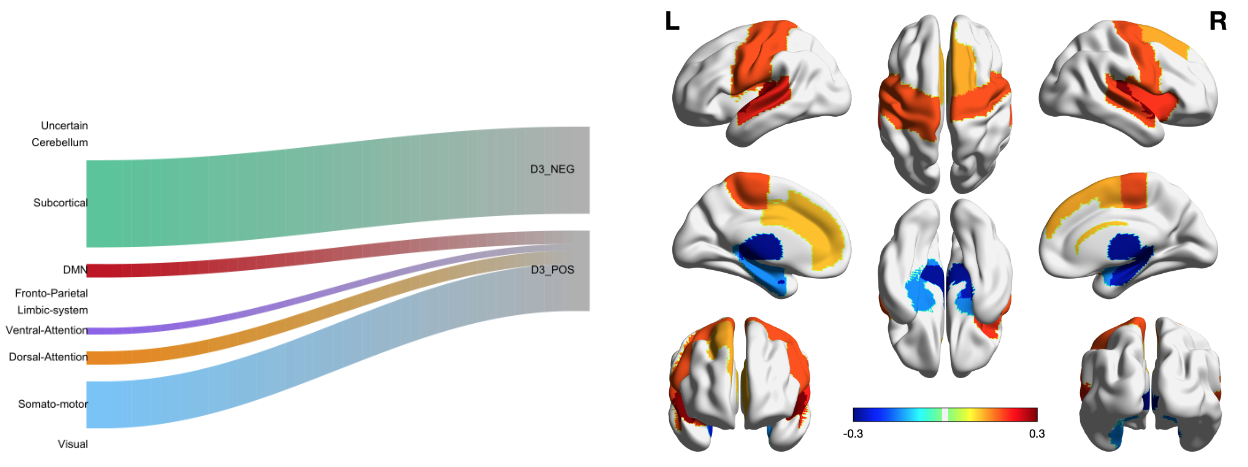Groups of regions with more or less connections predicted by APOE-$\varepsilon$4 (non)-carriers

## Discussion

• Regress PD matrices on vectors
• Method to identify covariate-related (supervised) directions vs (unsupervised) PCA
• Theorectical justifications
• Papers: Biostatistics (10.1093/biostatistics/kxz057), EJS (10.1214/21-EJS1887), Brain Behv (10.1002/brb3.1942)
• R pkg: cap